Contents >> Applied Mathematics >> Numerical Methods >> Algebraic and Transcendental Equations >> Method of halving

 Algebraic and transcendental equations - Method of halving Method of halving Let's consider the equation (1):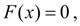where F ( x ) – is a continuous function, defined in the segment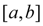and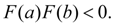The last means, that function F ( x ) has into the segmentat least one root. Let's consider a case, when the root into the segmentis unique. We halve the segment. If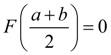, then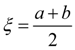is the root of the equation (1). If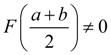, then we consider that half of the segmenton which ends function F ( x ) has different signs. The new, narrower segment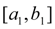we again halve and spent it on the same consideration and so on. As a result on some step we’ll receive either exact value of a root of the equation (1), or sequence of the segments enclosed each other: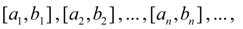such, that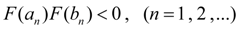(9) and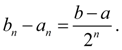(10) The left ends of these segments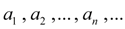form the monotonous (not decreasing) limited sequence, and the right ends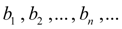– the monotonous (not increasing) limited sequence. Therefore by equality (10) there is a general limit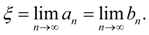Passing in (9) to a limit at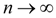, by continuity of the function F ( x ) we’ll receive: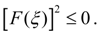Hence,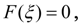that is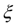is a root of the equation (1). In practice the process (10) is considered completed, if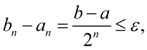(11) where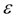– the given accuracy of solution.

 < Previous Contents Next >